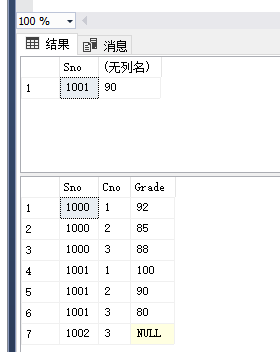# 例3.19到3.48

• 时间:
• 浏览:

## 数据查询

[例题3.19]

``````SELECT Sname,2021-Ssage
FROM Student;
``````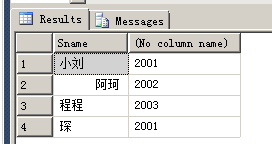[例题3.20]

``````SELECT Sname,'Year of birth:',2021-Ssage
FROM Student;
``````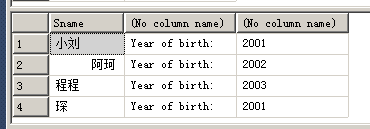``````SELECT Sname,'Year of birth:'BIRTH,2021-Ssage BIRTHDAY
FROM Student;
``````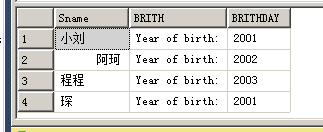【例3.21】消除重复的行

``````SELECT Sno
FROM SC;
``````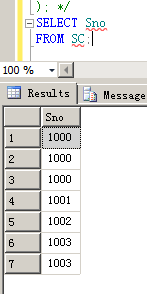``````SELECT DISTINCT Sno
FROM SC;
``````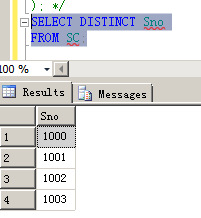【例3.22】查询计科全体学生名单

``````SELECT Sname
FROM Student
WHERE　Sdept='计科';
``````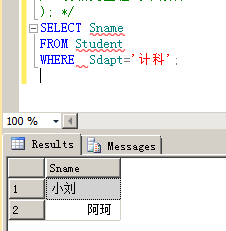【例3.23】查询年龄20岁以下的学生姓名和年龄

``````SELECT Sname，Sage
FROM Student
WHERE　Sage<20;
``````

【例3.24】查询成绩不及格的

``````select distinct Sno
from SC
where Grade<60;
``````

【例3.25】between and 使用

``````select Sname,Sdept,Sage
from Student
where Sage between 20 and 23;
``````

【例3.26】not between and

``````select Sname,Sdept,Sage
from Student
where Sage not between 20 and 23;
``````

【例3.27】【例3.28】略

【例3.29】查询学号为1001的学生情况

``````select *
from Student
where Sno like'1001';

``````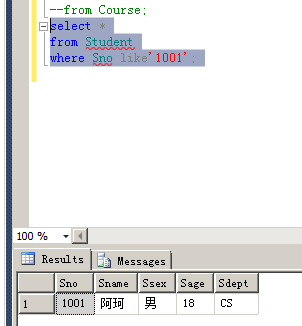【例3.30】查询姓狗的学生

``````select *
from Student
where Sname like'狗%';
``````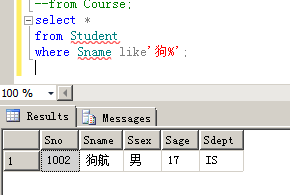【例3.31】查询姓小且名字只有两个字的人

``````select *
from Student
where Sname like'小_';
``````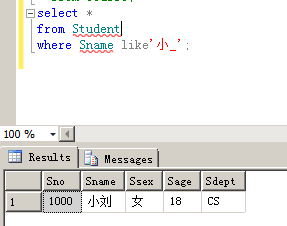``````select *
from Student
where Sname like'小__';
``````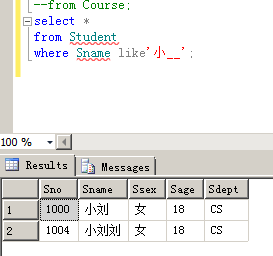``````select *
from Student
where Sname like'小__' And Sname NOT LIKE '小_';
``````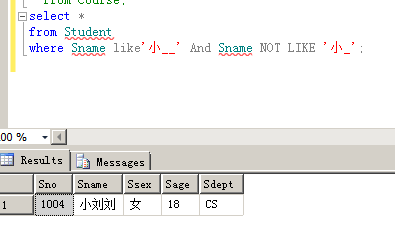【例3.34】查DB_Design课程的相关信息

``````select *
from Course
where Cname like'DB_Design';
``````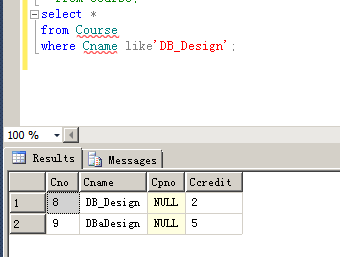``````select*
from Course
where Cname like'DB\_Design'escape'\';
``````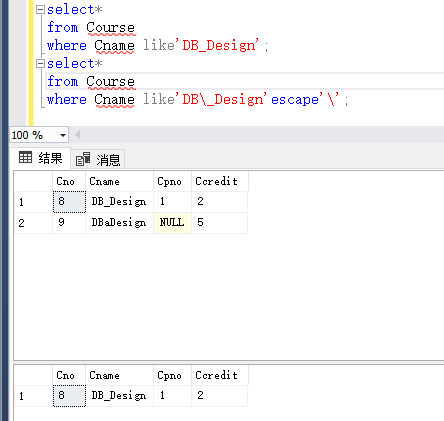【例3.36】涉及空值的查询

``````select*
from SC
where Grade is NULL;
select*
from SC
where Grade is not NULL;
select*
from SC
where Grade = NULL;
``````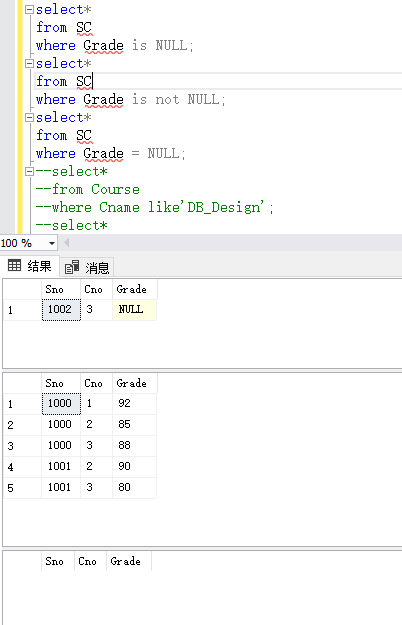【例3.38】查询计科系20岁以下的学生姓名（and的使用）

``````select Sname
from Student
where Sage<20 and Sdept='CS';
``````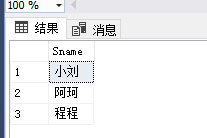order by句子的使用：

【例3.39】查询选修了3号课程的学生的成绩并按照降序排序

``````select Sno, Grade
from SC
where Cno='3 '
order by Grade DESC;
``````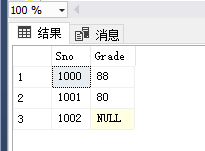count（*）：统计元组个数
count（[distinct|all]<列名>）：统计一组中值的个数
sum（[distinct|all]<列名>）：计算一列值的总和（此列必须为数值型）
avg（[distinct|all]<列名>）：计算一列值的平均值（此列必须为数值型）
max（[distinct|all]<列名>）：求一列中最大值
min（[distinct|all]<列名>）：求一列中最小值
【例3.41】查学生总人数

``````select count(*)
from Student;
``````

【例3.42】查询选修了的学生人数

``````select count(distinct Sno)
from SC;
``````

【例3.43】查询选修了一号课程的学生的平均成绩

``````select avg(grade）
from SC
where Cno='1';
``````

【例3.44】查询选修了一号课的学生的最高分

``````select max(grade)
from SC
where Cno='1';
``````

【例3.45】查询学生1001选修课程的总分

``````selest sum(grade)
from SC Course
where Sno='1001' and SC.Cno=Course.Cno;
``````

group by子句：该子句将查询结果按某一列或多列的值进行分组，值相等的为一组。
【例3.46】求各个课程号及相应的选课人数

``````select  Cno,Count(Cno)renshu
from SC
group by Cno;
``````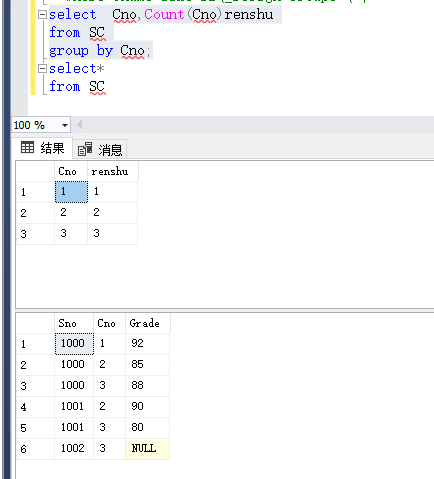【例3.47】查询选修了2门课及以上的学生学号

``````select  Sno
from SC
group by Sno
having count(*)>=2;
``````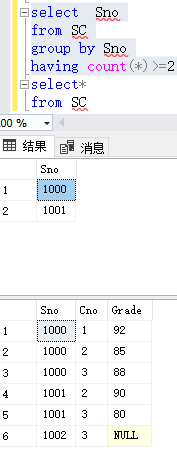where子句与having子句的作用对象不同，where子句后面不可以接聚集函数而having可以接、
【例3.48】查询平均成绩大于等于90分的学生学号和平均成绩

``````select Sno,avg(Grade)
from SC
where avg(Grade)>=90
group by Sno;
``````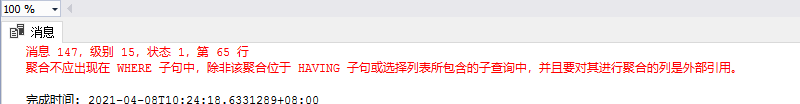``````select Sno,avg(Grade)
from SC
group by Grade
having avg(Grade)>=90;
``````